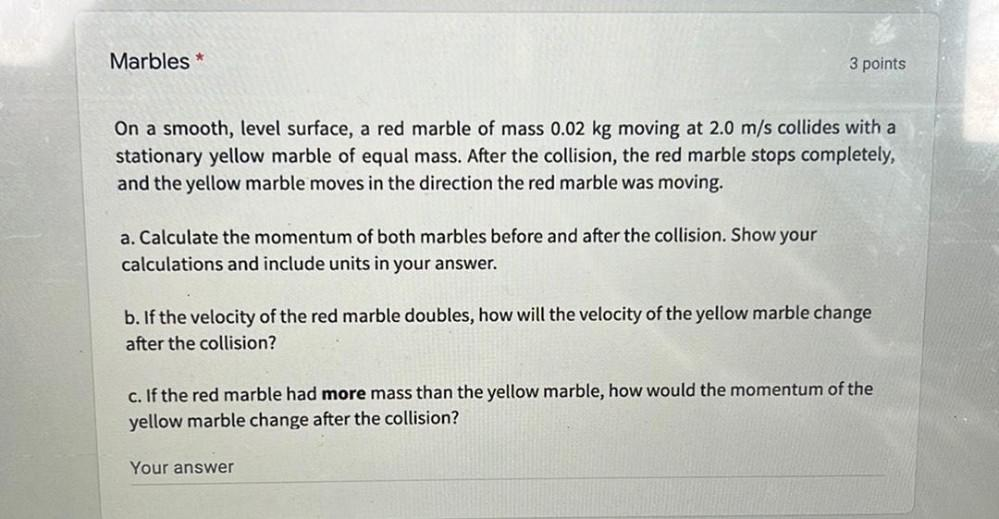Question:

# Marbles * 3 points On a smooth, level surface, a red marble of mass 0.02 kg moving at 2.0 m/s collides with a stationary yellowMarbles * 3 points On a smooth, level surface, a red marble of mass 0.02 kg moving at 2.0 m/s collides with a stationary yellow marble of equal mass. After the collision, the red marble stops completely, and the yellow marble moves in the direction the red marble was moving. a. Calculate the momentum of both marbles before and after the collision. Show your calculations and include units in your answer. b. If the velocity of the red marble doubles, how will the velocity of the yellow marble change after the collision? c. If the red marble had more mass than the yellow marble, how would the momentum of the yellow marble change after the collision? Your answer